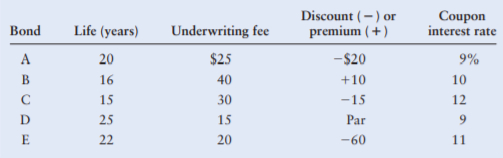### Create an Account

Already have account?

### Forgot Your Password ?

Home / Questions / Cost of debt using the approximation formula For each of the following 1000 parvalue bonds...

# Cost of debt using the approximation formula For each of the following 1000 parvalue bonds assuming annual interest payment and a 40 tax rate calculate the after-tax cost to maturity using the

Cost of debt using the approximation formula For each of the following \$1,000-parvalue bonds, assuming annual interest payment and a 40% tax rate, calculate the after-tax cost to maturity using the approximation formula.Jun 03 2021 View more View Less

#### Answer (UnSolved)Get Solution